# Blogs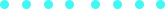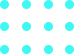### Look up! The Cook-Torrance!

Published on Mar 28, 2019 by on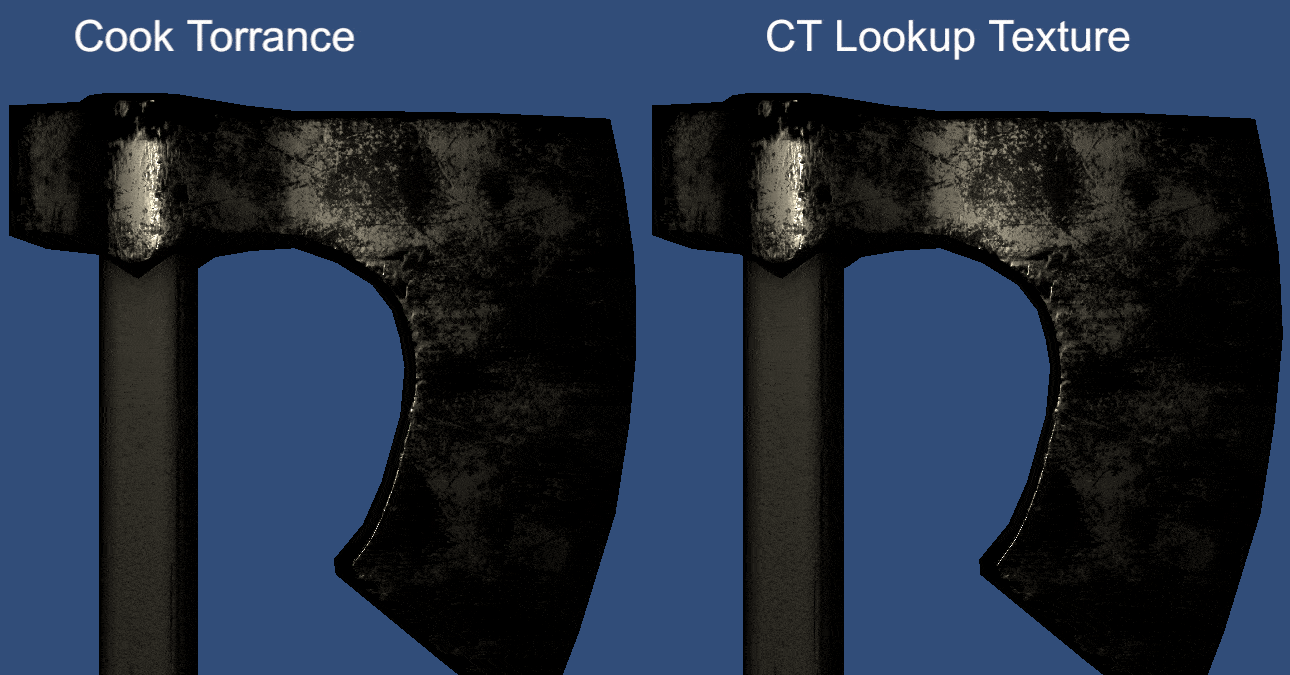Continuing the posts of stuff that I should have posted last year but for some reason didn’t. Here, some (not deep) thoughts on Cook-Torrance and lookup textures.

Cook-Torrance is a BRDF (Bidirectional Reflectance Distribution Function) broadly used in engines for specular reflection. In Claudia Doppioslash’s book, you can find an implementation similar to the following, with minor changes.

``````float sqr(float value)
{
return value * value;
}

float G1(float k, float x)
{
return x / (x * (1 - k) + k);
}

float CookTorranceSpec(float NdotL, float LdotH, float NdotH, float NdotV, float roughness, float f0)
{
float alpha = sqr(roughness);

float alphaSqr = sqr(alpha);
float denominator = sqr(NdotH) * (alphaSqr - 1.0) + 1.0;
float d = alphaSqr / (PI * sqr(denominator));

float f = f0 + (1 - f0) * pow(1 - LdotH, 5);

float modifiedRoughness = roughness + 1;
float k = sqr(modifiedRoughness) * 0.125;

float g1L = G1(k, NdotL);
float g1V = G1(k, NdotV);

float g = g1L * g1V;

return NdotL * d * g * f;
}
``````

I have this problem that makes me look at shader code and think ‘well, this could be baked into a texture’. Do I have a good reason for doing so? Usually not. Is this useful? Sometimes. I just can’t resist to those four channels looking at me saying ‘hey, put some data in here’, ‘I might be useful somehow’.

The easiest way of baking data into a texture is simply to store the result of two-dimensional functions in there, where the input values are normalized. If your functions return scalar values, then you have up to four channels to store results (i.e. four functions). In the case of the Cook-Torrance, four functions can be defined:

• F(LdotH, Spec)
• G1L(Roughness, NdotL)
• G1V(Roughness, NdotV)
• D(Roughness, NdotH)

Note that all inputs are in the `[0, 1]` range. Then, we can precompute these functions and store the results in the channels of our lookup texture. And we will actually need only three channels since G1L and G1V represent the same function.

The following code precomputes and stores the values in the appropriate channels. In this case, the lookup texture has to be a `RGBAFloat` texture because we will need to store high range values (and it has to be exported as .exr as well).

``````for (int x = 0; x < width; ++x)
{
float xNorm = (float) x / (width - 1);
float roughness = xNorm;
float alpha = roughness * roughness;
float alphaSqr = alpha * alpha;
float k = roughness + 1;
k = k * k * 0.125f;

float LdotH5 = Mathf.Pow(1 - xNorm, 5);

// R -> F -> LdotH x Spec
// G -> G -> Roughness x NdotL
// G -> G -> Roughness x NdotV
// B -> D -> Roughness x NdotH

for (int y = 0; y < height; ++y)
{
float yNorm = (float)y / (height - 1);

float F = yNorm + (1 - yNorm) * LdotH5;

float denom = yNorm * yNorm * (alphaSqr - 1) + 1;

denom = Mathf.Max(Mathf.PI * denom * denom, 0.0000001f);
float D = alphaSqr / denom;

float G = yNorm / Mathf.Max(yNorm * (1 - k) + k, 0.0000001f);

Color c = new Color(F, G, D, 0);
lut.SetPixel(x, y, c);
}
}
``````

The texture generated will look like this (the original can be found here)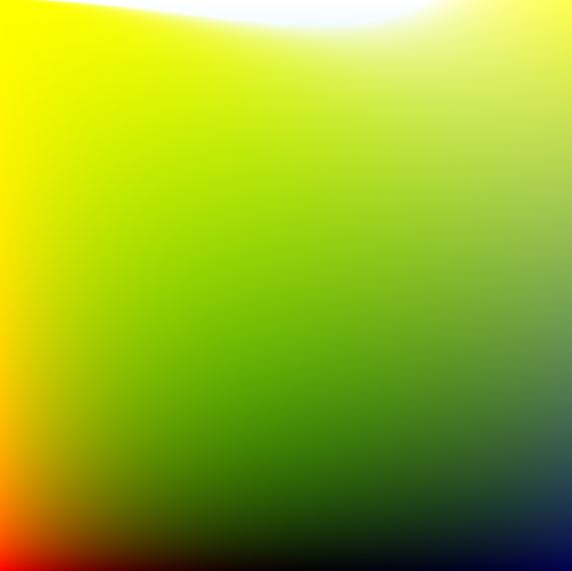With this lookup texture, we can then replace the `CookTorranceSpec(...)` call in our shader by the following code.

``````float F = tex2D(_CookTorranceLUT, float2(LdotH, 0.04)).r;
float G1L = tex2D(_CookTorranceLUT, float2(roughness, NdotL)).g;
float G1V = tex2D(_CookTorranceLUT, float2(roughness, NdotV)).g;
float D = tex2D(_CookTorranceLUT, float2(roughness, NdotH)).b;

float spec = NdotL * F * G1L * G1V * D;
``````

## Results

(Unity Standard x Cook-Torrance with Lookup Texture)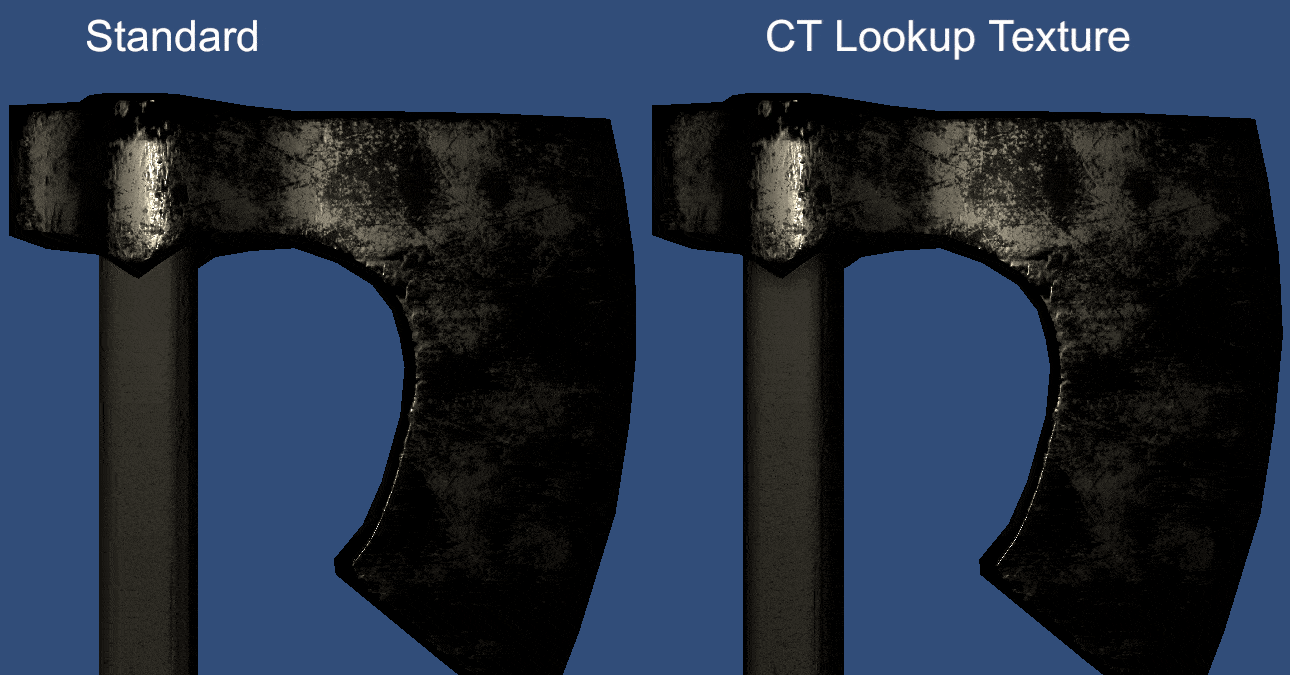(Cook-Torrance x Cook-Torrance with Lookup Texture)## Discussion

Is it worth it? It depends. The shader, in my example, that computes the Cook-Torrance at runtime has 53 math operations, 3 temp registers and 3 textures (fragment shader), whereas the version with lookup texture has 35 math, 6 temp registers, 7 textures. By using the lookup texture, we save operations, but increase samplers. We will also end up with less precise results (you will need to find a tradeoff between the acceptable visuals and the lookup texture resolution). If you are working with mobile, it is definitely worth trying this and maybe trying to make some adaptations (in some cases, you might remove the geometric function (G1V and G1L) and still have plausible results).

That’s it, for now. If I talked too much nonsense please correct me on twitter, I’d appreciate that.

All the code is available here

## Similar Stories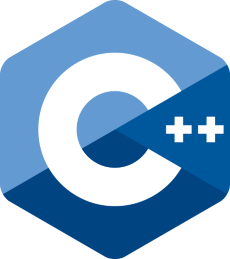#### Annileen Devlog #2 - C++20 and Modules#### From Built-in to URP

Unity’s Scriptable Render Pipeline represents a great advance on the way that unity deals with graphics, giving more power to the users to customize the pipeline the way they want....#### Look up! The Cook-Torrance!

Continuing the posts of stuff that I should have posted last year but for some reason didn’t. Here, some (not deep) thoughts on Cook-Torrance and lookup textures.